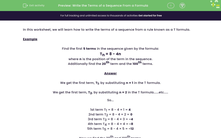# Write the Terms of a Sequence From a Formula

In this worksheet, students find the first few terms of a sequence from the given T formulae.Key stage:  KS 3

Curriculum topic:   Algebra

Curriculum subtopic:   Generate Terms of a Sequence

Difficulty level:#### Worksheet Overview

In this worksheet, we will learn how to write the terms of a sequence from a rule known as a T formula.

Example

Find the first 5 terms in the sequence given by the formula:

Tn = 8 - 4n

where n is the position of the term in the sequence.

Additionally find the 20th term and the 100th terms.

We get the first term, T1, by substituting n = 1 in the T formula.

We get the first term, T2, by substituting n = 2 in the T formula......etc.....

So...

1st term T1 = 8 - 4 × 1 = 4
2nd term T2 = 8 - 4 × 2 = 0
3rd term T3 = 8 - 4 × 3 = -4
4th term T4 = 8 - 4 × 4 = -8
5th term T5 = 8 - 4 × 5 = -12

Now we find the 20th and 100th terms.

T20 = 8 - 4 × 20 = 8 - 80 = -72
T100 = 8 - 4 × 100 = 8 - 400 = -392

### What is EdPlace?

We're your National Curriculum aligned online education content provider helping each child succeed in English, maths and science from year 1 to GCSE. With an EdPlace account you’ll be able to track and measure progress, helping each child achieve their best. We build confidence and attainment by personalising each child’s learning at a level that suits them.

Get started